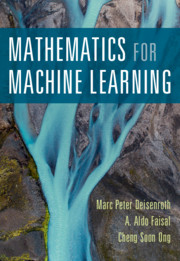## Free Ebook: Mathematics for Machine Learning# Free Ebook: Mathematics for Machine Learning

Jupyter Notebook: https://github.com/mml-book/mml-book.github.io

Part I: Mathematical Foundations

1. Introduction and Motivation
2. Linear Algebra
3. Analytic Geometry
4. Matrix Decompositions
5. Vector Calculus
6. Probability and Distribution
7. Continuous Optimization

Part II: Central Machine Learning Problems

1. When Models Meet Data
2. Linear Regression
3. Dimensionality Reduction with Principal Component Analysis
4. Density Estimation with Gaussian Mixture Models
5. Classification with Support Vector Machines